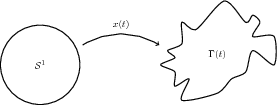# Parametric Setting

In order to solve the coupled system analytically or numerically, we need to give a description of our surface. There are several approaches we could take; parametric, graph, level set, phase field. We will consider the first two methods.

• Parametric approach - Mesh point will evolve with normal velocity leading to mesh degeneration. We aim to resolve this issue with the DeTurck trick.
• Graph approach - Although this assumption is restrictive in terms of modelling, it will enable us to prove local existence. The ideas and techniques will be a useful tool in understanding the general situation.

We parametrise the evolving closed curve$\Gamma(t) \subset \mathbb{R}^2$ using a stationary reference manifold,$\Gamma(t) = x(S^1,t) , \qquad x:\mathcal{S}^1\times[0,T)\to\mathbb{R}^2.$Mesh degeneration

A key problem with this approach is that our mesh points move with normal velocity resulting in mesh degeneration. As a result, this will produce large errors in our numerical schemes. To resolve this issue we will use the DeTurck trick, which is a reparametrisation that introduces tangential motion.

Forced curve shortening flow with the DeTurck trick

Applying the DeTurck reparametrisation to curve shortening flow with forcing, we have$\partial_t \hat{x}_\alpha=(-\kappa+f)\, \nu\circ \hat{x}_\alpha -\tfrac{1}{\alpha} \, d\hat{x}_\alpha(V_\alpha)$

In local coordinates the equation becomes:$\alpha \hat{X}_{\alpha, t} + (1-\alpha)(\nu \cdot \hat{X}_{\alpha,t}) \, \nu = |\hat{X}_{\alpha, \theta \theta}|^{-2} \, \hat{X}_{\alpha,\theta\theta} + f(\hat{X}_{\alpha}) \, \nu$
Semi-discrete scheme

Discretising the spatial variables using linear finite elements we obtain the semi-discrete scheme$\int_0^{2\pi} \big{(} \alpha \hat{X}_{ht} \cdot \phi_h + (1-\alpha)(\nu_h\cdot \hat{X}_{ht})(\nu_h\cdot \phi_h) \big{)} |\hat{X}_{h\theta}|^2 + \hat{X}_{h\theta} \cdot \phi_{h\theta} \, d\theta = \int_0^{2\pi} |\hat{X}_{h\theta}|^2 \, f(\hat{X}_{h}) \, \nu_h \cdot \phi_h \, d\theta.$

A suitable convergence result can be proved, however the constant in the error estimate will blow up as$\alpha \rightarrow 0$. The problem of convergence with$\alpha = 0$ remains open.